NEET  >  Test: Waves - 4

# Test: Waves - 4

Test Description

## 25 Questions MCQ Test Physics Class 11 | Test: Waves - 4

Test: Waves - 4 for NEET 2023 is part of Physics Class 11 preparation. The Test: Waves - 4 questions and answers have been prepared according to the NEET exam syllabus.The Test: Waves - 4 MCQs are made for NEET 2023 Exam. Find important definitions, questions, notes, meanings, examples, exercises, MCQs and online tests for Test: Waves - 4 below.
Solutions of Test: Waves - 4 questions in English are available as part of our Physics Class 11 for NEET & Test: Waves - 4 solutions in Hindi for Physics Class 11 course. Download more important topics, notes, lectures and mock test series for NEET Exam by signing up for free. Attempt Test: Waves - 4 | 25 questions in 50 minutes | Mock test for NEET preparation | Free important questions MCQ to study Physics Class 11 for NEET Exam | Download free PDF with solutions
 1 Crore+ students have signed up on EduRev. Have you?
*Multiple options can be correct
Test: Waves - 4 - Question 1

### To observe a stationary interference pattern formed by two light waves, it is not necessary that they must have :

*Multiple options can be correct
Test: Waves - 4 - Question 2

### In a YDSE apparatus, we use white light then :

*Multiple options can be correct
Test: Waves - 4 - Question 3

### If the source of light used in a Young's Double Slit Experiment is changed from red to blue, then

*Multiple options can be correct
Test: Waves - 4 - Question 4

In a Young's double slit experiment, green light is incident on the two slits. The interference pattern is observed on a screen. Which of the following changes would cause the observed fringes to be more closely spaced ?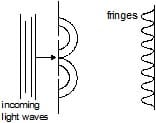*Multiple options can be correct
Test: Waves - 4 - Question 5

In a Young's double-slit experiment, let A and B be the two slits. A thin film of thickness t and refractive index m is placed in front of A. Let b = fringe width. The central maximum will shift :

*Multiple options can be correct
Test: Waves - 4 - Question 6

In the previous question, films of thicknesses tA and tB and refractive indices mA and mB, are placed in front of A and B respectively. If mAtA = mBtB, the central maximum will :

*Multiple options can be correct
Test: Waves - 4 - Question 7

In a YDSE, if the slits are of unequal width.

*Multiple options can be correct
Test: Waves - 4 - Question 8

If one of the slits of a standard YDSE apparatus is covered by a thin parallel sided glass slab so that it transmit only one half of the light intensity of the other, then

*Multiple options can be correct
Test: Waves - 4 - Question 9

To make the central fringe at the centre O, a mica sheet of refractive index 1.5 is introduced. Choose the correct statements (s).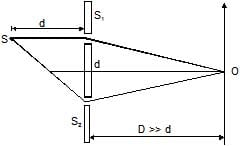*Multiple options can be correct
Test: Waves - 4 - Question 10

Choose the correct statement(s) related to the wavelength of light used

*Multiple options can be correct
Test: Waves - 4 - Question 11

If the distance D is varied, then choose the correct statement(s)

*Multiple options can be correct
Test: Waves - 4 - Question 12

If the distance d is varied, then identify the correct statement

*Multiple options can be correct
Test: Waves - 4 - Question 13

In an interference arrangement similar to Young's double-slit experiment, the slits S1 & S2 are illuminated with coherent microwave sources, each of frequency 106 Hz. The sources are synchronized to have zero phase difference. The slits are separated by a distance d = 150.0 m. The intensity I(q) is measured as a function of q, where q is defined as shown. If I0 is the maximum intensity then I(q) for 0 £ q £ 90° is given by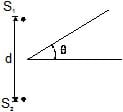Detailed Solution for Test: Waves - 4 - Question 13

I=I0cos2(πdtanθ/ λ)/
= I0cos2(πx150xtanθ/3x(108/106)
=I0cos2(πtanθ/2)
θ=30o
I= I0cos2 ((π/2√3)
I=I0/2
θ=0
I=I0cos2(0) [cos0=1]
I= I0

*Multiple options can be correct
Test: Waves - 4 - Question 14

In a standard YDSE appratus a thin film (m = 1.5, t = 2.1 mm) is placed in front of upper slit. How far above or below the centre point of the screen are two nearest maxima located ? Take D = 1 m, d = 1mm, l = 4500 Å. (Symbols have usual meaning)

Detailed Solution for Test: Waves - 4 - Question 14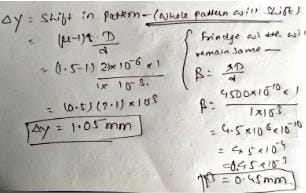*Multiple options can be correct
Test: Waves - 4 - Question 15

Consider a case of thin film interference as shown. Thickness of film is equal to wavelength of light is m2.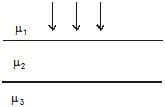*Multiple options can be correct
Test: Waves - 4 - Question 16

Figure shown plane waves refracted for air to water using Huygen's principle a, b, c, d, e are lengths on the diagram. The refractive index of water wrt air is the ratio.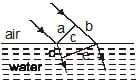Detailed Solution for Test: Waves - 4 - Question 16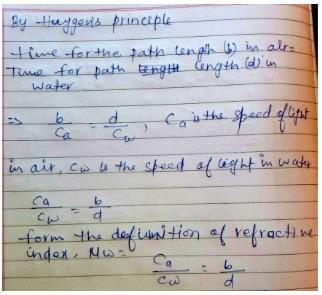Test: Waves - 4 - Question 17

Two point source separated by

d = 5mm emit light of wavelength l = 2mm in phase. A circular wire of radius 20mm is placed around

the source as shown in figure.

Test: Waves - 4 - Question 18

Two monochromatic (wavelength = a/5) and coherent sources of electromagnetic waves are placed on the x-axis at the points (2a, 0) and (_a, 0). A detector moves in a circle of radius R(>>2a) whose centre is at the origin. The number of maximas detected during one circular revolution by the detector are

Test: Waves - 4 - Question 19

Two coherent narrow slits emitting light of wavelength l in the same phase are placed parallel to each other at a small separation of 3l. The light is collected on a screen S which is placed at a distance D (>> l) from the slits. The smallest distance x such that the P is a maxima.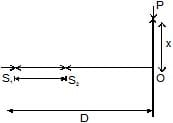Test: Waves - 4 - Question 20

A beam of light consisting of two wavelengths 6500 Å and 5200 Å is used to obtain interference fringes in Young's double slit experiment. The distance between slits is 2mm and the distance of screen from slits is 120 cm. What is the least distance from central maximum where the bright due to both wavelength coincide ?

Detailed Solution for Test: Waves - 4 - Question 20

If n is least number of fringes of λ1(=6500A∘) which are coincident with (n+1) of smaller wavelength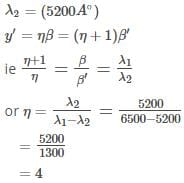also β=λD/d
substituting values for wavelength,D and d we get β = 0.039cm
So,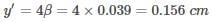Hence b is the correct answer.

Test: Waves - 4 - Question 21

In Young's double slit experiment, the two slits act as coherent sources of equal amplitude A and wavelength l. In another experiment with the same setup the two slits are sources of equal amplitude A and wavelength l but are incoherent. The ratio of the average intensity of light at the midpoint of the screen in the first case to that in the second case is

Test: Waves - 4 - Question 22

A monochromatic light source of wavelength l is placed at S. Three slits S1, S2 and S3 are equidistant from the source S and the point P on the screen. S1P _ S2P = l/6 and S1P _ S3P = 2l/3. If I be the intensity at P when only one slit is open, the intensity at P when all the three slits are open is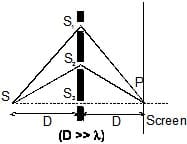Test: Waves - 4 - Question 23

In the figure shown in YDSE, a parallel beam of light is incident on the slit from a medium of refractive index n1. The wavelength of light in this medium is l1. A transparent slab to thickness `t' and refractive index n3 is put infront of one slit. The medium between the screen and the plane of the slits is n2. The phase difference between the light waves reaching point `O' (symmetrical, relative to the slits) is :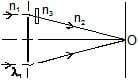Test: Waves - 4 - Question 24

In a YDSE experiment if a slab whose refractive index can be varied is placed in front of one of the slits then the variation of resultant intensity at mid-point of screen with `m' will be best represented by (m ³ 1). [Assume slits of equal width and there is no absorption by slab]

Test: Waves - 4 - Question 25

In a YDSE with two identical slits, when the upper slits is covered with a thin, perfectly transparent sheet of mica, the intensity at the centre of screen reduces to 75% of the initial value. Second minima is observed to be above this point and third maxima below it. Which of the following can not be a possible value of phase difference caused by the mica sheet.

## Physics Class 11

127 videos|464 docs|210 tests
 Use Code STAYHOME200 and get INR 200 additional OFF Use Coupon Code
Information about Test: Waves - 4 Page
In this test you can find the Exam questions for Test: Waves - 4 solved & explained in the simplest way possible. Besides giving Questions and answers for Test: Waves - 4, EduRev gives you an ample number of Online tests for practice

## Physics Class 11

127 videos|464 docs|210 tests

### How to Prepare for NEET

Read our guide to prepare for NEET which is created by Toppers & the best Teachers